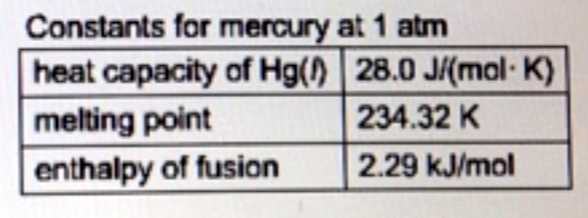# Problem: Calculate the heat energy (kJ) released when 23.6 g of liquid mercury at 25.00°C is converted to solid mercury at its melting point.

🤓 Based on our data, we think this question is relevant for Professor Culbertson's class at K- STATE.

###### FREE Expert Solution

We’re being asked to calculate the amount of energy released when 23.6 g of mercury at 25.00 ˚C is converted to a solid at its melting point (234.32 K or –38.68 ˚C).

Note that cooling and freezing is an exothermic process, which means q is negative (–)

There are two heats involved in this problem:

1. q1 which is the heat in cooling 23.6 g of Hg from 25 ˚C to –38.68 ˚C

2. q2 which is the heat in freezing 23.6 g of Hg at –38.68 ˚C

We need to solve for each heat individually then add them together to get the final answer.###### Problem Details

Calculate the heat energy (kJ) released when 23.6 g of liquid mercury at 25.00°C is converted to solid mercury at its melting point.